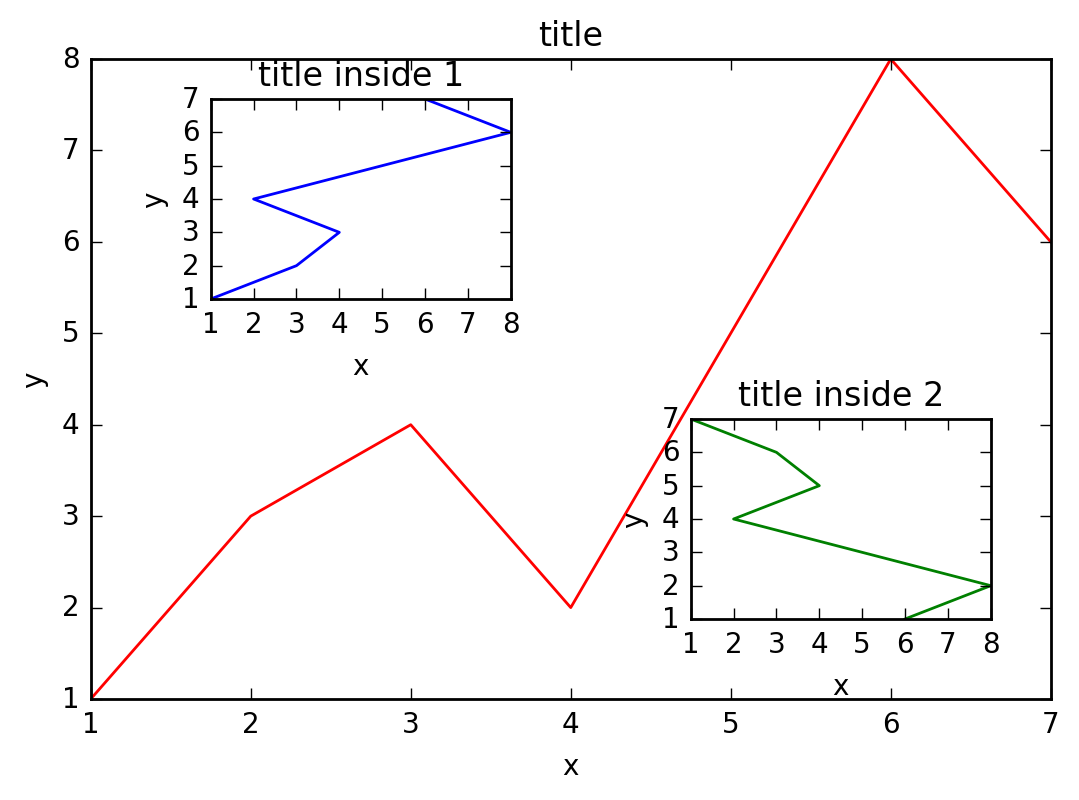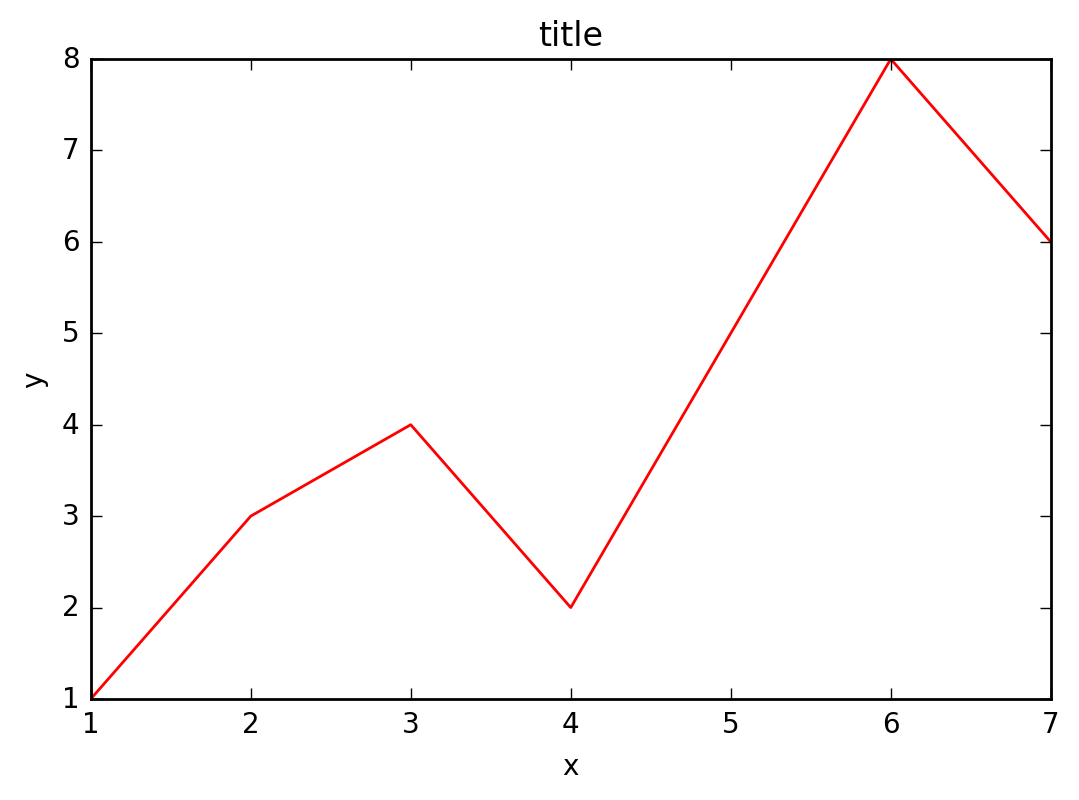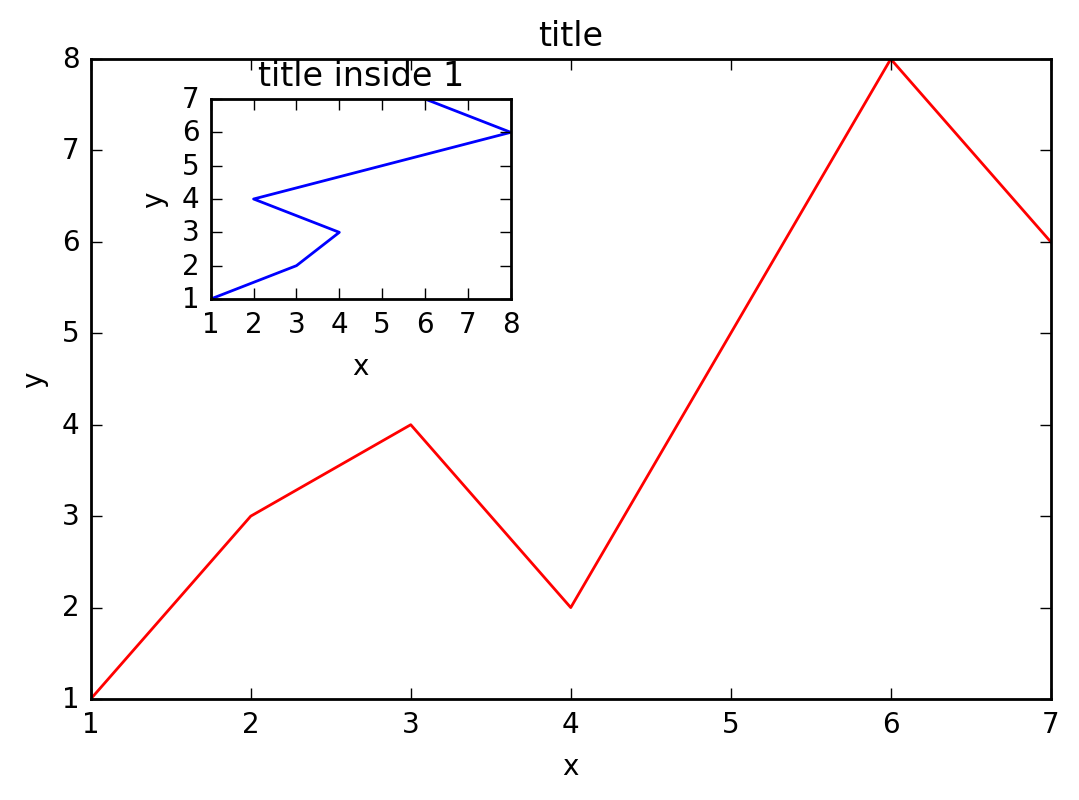# Plot Inside Plot With Matplotlib

this is not my article, this is just English translation of: 图中图 | 莫烦Python

This time we will talk about a very interesting function in matplotlib called plot in plot. The final effect is as follows:It can be seen that the whole Figure 1 contains three pictures, two of which appear in the small picture `title inside 1`and the big picture . How is this done?`title inside 2``title`

# data¶

First some preparations:

``````import matplotlib.pyplot as plt

fig = plt.figure()

# generate data to plot
x = [1, 2, 3, 4, 5, 6, 7]
y = [1, 3, 4, 2, 5, 8, 6]
``````

# Big

Next, let's draw the big picture. First determine the position, width, and height of the lower left corner of the large image:

``````left, bottom, width, height = 0.1, 0.1, 0.8, 0.8
``````

Note that the 4 values ​​are `figure`all percentages of the entire coordinate system. Here, assuming `figure`that the size is 10x10, then the large image is included in the coordinate system starting from (1, 1), 8 in width, and 8 in height.

Add the large image coordinate system to `figure`it, the color is r (red), and the name is title:

``````ax1 = fig.add_axes([left, bottom, width, height])
ax1.plot(x, y, 'r')
ax1.set_xlabel('x')
ax1.set_ylabel('y')
ax1.set_title('title')
``````

The effect is as follows:# Thumbnails

Next, let's draw the small picture in the upper left corner, the steps are the same as drawing the big picture, pay attention to the change of the position and size of the coordinate system:

``````left, bottom, width, height = 0.2, 0.6, 0.25, 0.25
ax2 = fig.add_axes([left, bottom, width, height])
ax2.plot(y, x, 'b')
ax2.set_xlabel('x')
ax2.set_ylabel('y')
ax2.set_title('title inside 1')
``````

The effect is as follows:Finally, let's draw the small graph in the lower right corner. Here we adopt a simpler method, that is, add a new coordinate system directly to plt:

``````plt.axes([0.6, 0.2, 0.25, 0.25])
plt.plot(y[::-1], x, 'g')
plt.xlabel('x')
plt.ylabel('y')
plt.title('title inside 2')
``````

Finally display the image:

``````plt.show()
``````Credits: all credits goes to original author: Mofan Zhou (this page is just a translation)